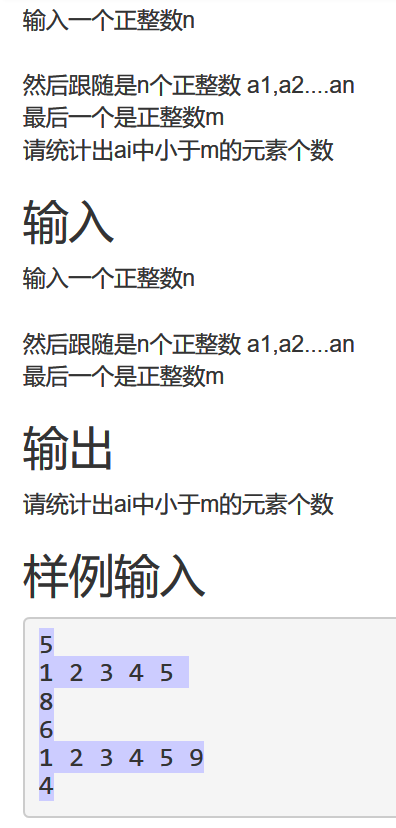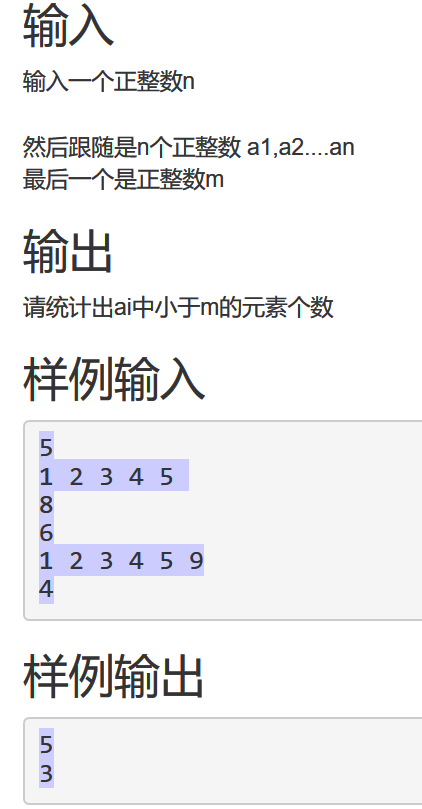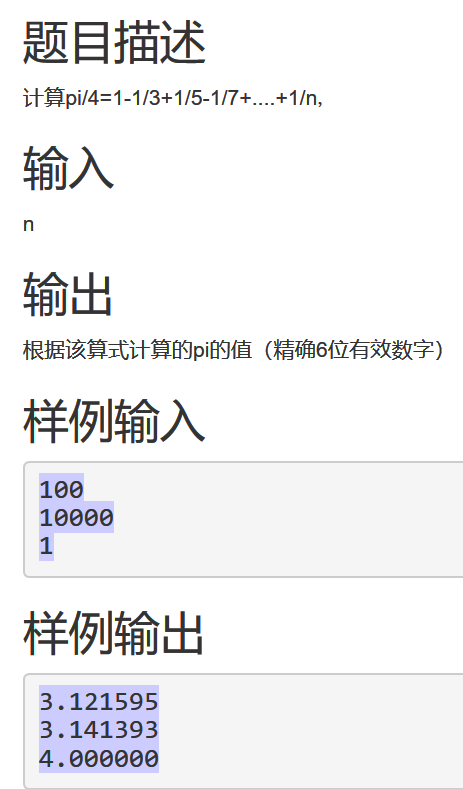2018-10-21 14:00

# 大一新生，跪求大神解答，作业每天就要提交了，真心急，谢谢• 点赞
• 写回答
• 关注问题
• 收藏
• 邀请回答

#### 2条回答默认 最新

•已采纳
`````` #include<iostream>
using namespace std;
int main(){
int n, m, count;
while (1) {                    //死循环
count = 0;
cin >> n;
int *list = new int[n];    //创建动态数组
//int list;         //刚学不会的话直接创建一个很大空间的数组吧
for (int i = 0; i < n; i++) {
cin >> list[i];
}
cin >> m;
for (int j = 0; j < n; j++) {
if (list[j] < m)
count++;
}
cout << count << endl;
}
return 0;
}

``````
`````` #include<iostream>
using namespace std;
int main(){
int n;
double pi,sum;
while (1) {      // 死循环
cin >> n;
sum = 0;
for (int i = 0; (2 * i + 1) <= n; i++) {
sum = sum + pow(-1, i) / (2 * i + 1); //pow为数学函数，这里是-1的i次方
}
pi = 4 * sum;
printf("%0.6lf\n", pi);      //题目要求精确6位小数，这里用C语言的格式化输出语句比较简单
}
return 0;
}
``````
点赞 打赏 评论
•devmiao 2018-10-21 14:17

你这个2个问题分开问，再开一个。然后点赞+采纳这个回答。然后完整帮你写。之前回答很多，写一个就半个小时，结果采纳都没有，姐姐不敢贸然回答了都。

点赞 打赏 评论